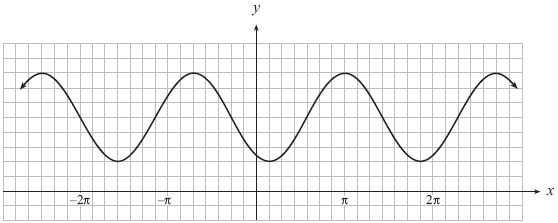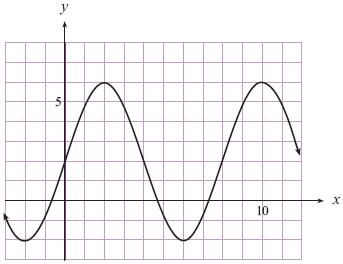# Determining trigonometric functions given their graphs#### Everything You Need in One Place

Homework problems? Exam preparation? Trying to grasp a concept or just brushing up the basics? Our extensive help & practice library have got you covered.#### Learn and Practice With Ease

Our proven video lessons ease you through problems quickly, and you get tonnes of friendly practice on questions that trip students up on tests and finals.#### Instant and Unlimited Help

Our personalized learning platform enables you to instantly find the exact walkthrough to your specific type of question. Activate unlimited help now!##### Examples
###### Lessons
1. For the following graph, write an equation (with the smallest phase shift) in the form of:
(i) y = a sin b(x - c) + d
(ii) y = - a sin b(x - c) + d
(iii) y = a cos b(x - c) + d
(iv) y = - a cos b(x - c) + d

1.2.2. A sinusoidal curve has a minimum point at $\left( { - \frac{\pi }{3},\; - 5} \right)$ and the closest maximum point to the right is $\left( {\;\frac{\pi }{6},\;3} \right)$. Determine an equation of this curve.